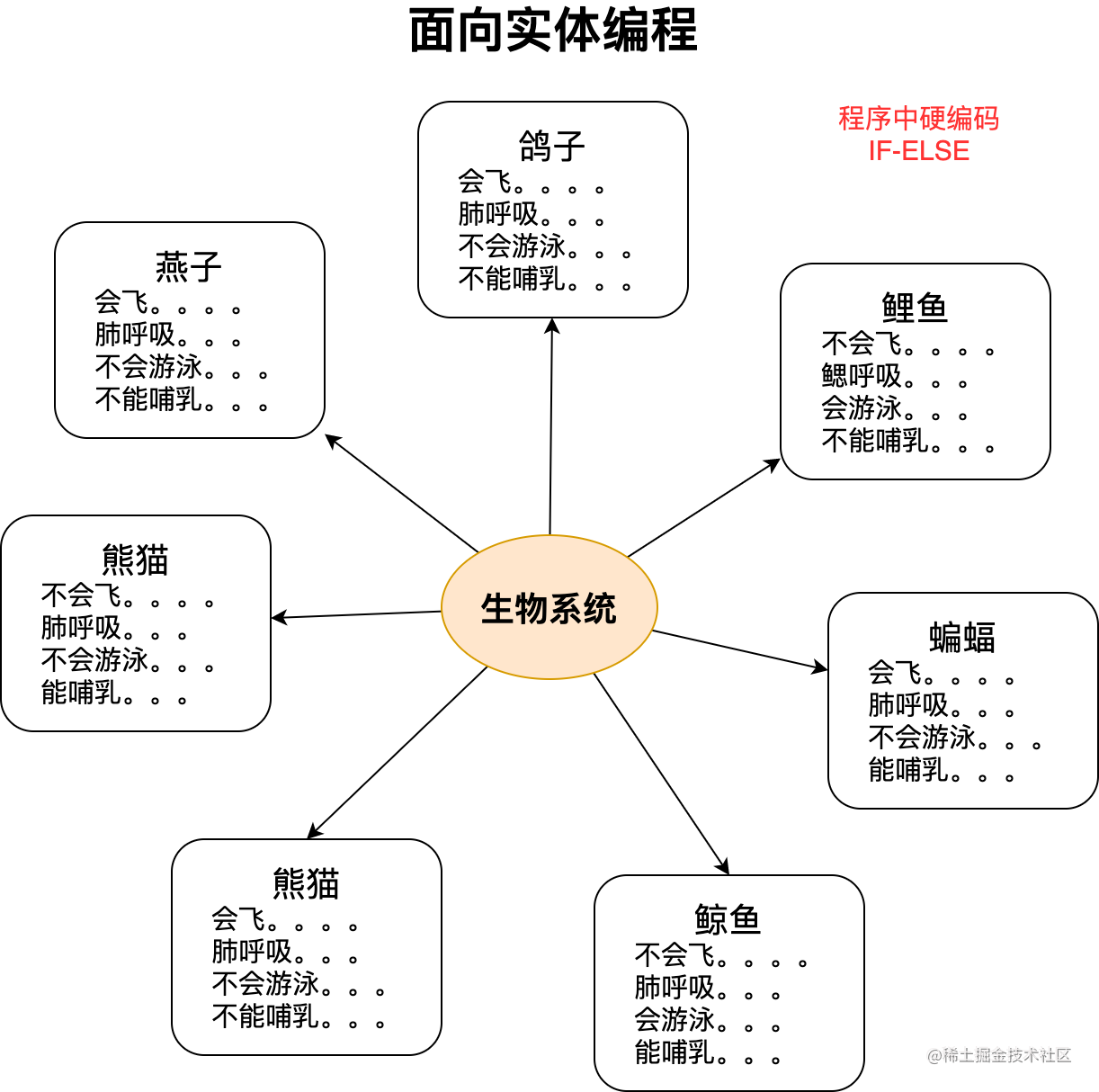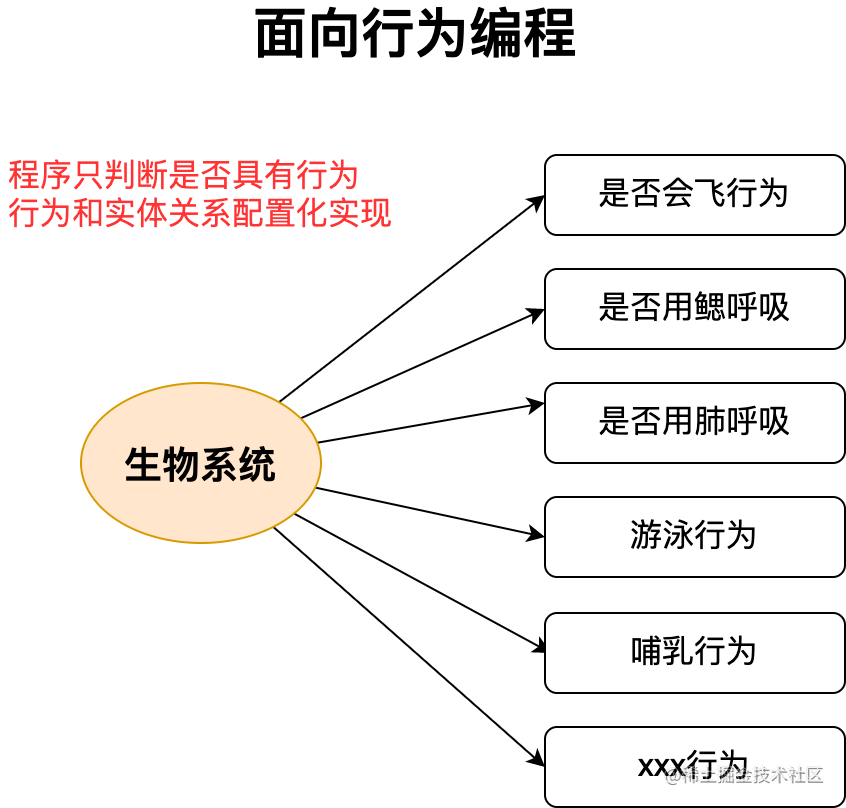# 使用面向行为编程解除项目耦合

``````if(需求A || 需求B){
doSometing1();
}
if(需求B){
doSometing2();
}
if(需求C || 需求F){
doSometing3();
}
if(需求A || 需求E){
doSometing4();
}
if(需求A || 需求M){
doSometing5();
}

``````if(燕子){
飞();
}
if(鲤鱼){
游泳（）;
}
if(燕子){
肺呼吸（）;
}
if(鲤鱼){
鳃呼吸（）;
}

``````if(燕子 || 鸽子 || 蝙蝠){
飞();
}
if(鲤鱼 || 鲸鱼){
游泳（）;
}
if(鸽子 || 蝙蝠 || 鲸鱼){
肺呼吸（）;
}
if(鲤鱼){
鳃呼吸（）;
}
if(蝙蝠 || 鲸鱼){
哺乳（）;
}``````if(能飞()){
飞();
}
if(能游泳()){
游泳（）;
}
if(能肺呼吸()){
肺呼吸（）;
}
if(能鳃呼吸){
鳃呼吸();
}
if(能哺乳()){
哺乳（）;
}